# Quick field calculator IF question

773
12
11-16-2017 02:44 AM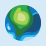Occasional Contributor II

Hi. I have a point FC with 2 text fields. 'Field 1' and 'Field 2'.

I want Field 2 to = 5 IF Field 1 = E

and also

Field 2 to = 4 IF Field 1 = D

So far I've tried this but it returned a syntax error in geoprocessing results:

if [field1] = 'E' then
result = "5"

Could I have any solutions in VB and python if possible? Will help me learn more!

Thanks!

ArcMap 10.5.1

Tags (1)
1 Solution

Accepted Solutions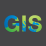byEsri Esteemed Contributor

Hi Theodore,

Using python, you can do this by right-clicking on field 2 > Calculate Field.   Check Python at the top and 'Show Code Block'.  Enter the following in the first block:

``````def calc(field):
if field == 'E':
return 5
elif field == 'D':
return 4‍‍‍‍‍``````

In the second code block enter:

``calc(!<field name>!)‍``

Where <field name> is the name of Field1.  When specifying something 'equal to' you will want to use == rather than just =.

12 RepliesbyEsri Esteemed Contributor

Hi Theodore,

Using python, you can do this by right-clicking on field 2 > Calculate Field.   Check Python at the top and 'Show Code Block'.  Enter the following in the first block:

``````def calc(field):
if field == 'E':
return 5
elif field == 'D':
return 4‍‍‍‍‍``````

In the second code block enter:

``calc(!<field name>!)‍``

Where <field name> is the name of Field1.  When specifying something 'equal to' you will want to use == rather than just =.Occasional Contributor II

Thanks, works perfectly!byMVP Esteemed Contributor

``````def cvt(field1):
if field1 == 'E':
out = 5
elif field1 == 'D':
out = 4
else:
out = -9
return out‍‍‍‍‍‍‍‍‍‍‍‍‍‍‍‍``````

In the field calculator, your Field2 needs to be an integer field.  That is where you are making the field calculation, so have it active, load the code block with

Parser.... python

expression.....   cvt(!Field!)

assuming your field1 is actually called Field1

It can be simplified, but I wanted to emulate your request with the addition of returning a -9 in the first two conditions were not metOccasional Contributor II

Thanks Dan,

Using your method my codeblock looks like:

def cvt(CLASS_RunS):
if CLASS_RunS == 'E':
out = 5
elif CLASS_RunS == 'D':
out = 4
else:
out = -9
return out

and my expression is:

cvt( !RunS_score!)

('CLASS_RunS' is the name of Field 1 which contains the Ds and Es. 'RunS_score' is the name of Field 2 where the 4s and 5s are to appear) was I meant to leave this as 'field' like in your reply?

I end up with everything being -9: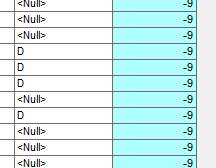Where have I gone wrong?

thanksbyMVP Esteemed Contributor

Ahhh you could have left my code as it was.

I assume that !RunScore! is the field containing the data and not the field you are trying to calculate, it is kindof truncated in your screen grabOccasional Contributor II

ah sorry: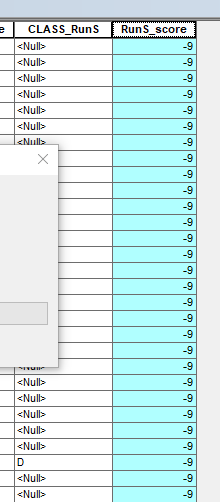No, RunS_score is the field I'm trying to calculate. Have I used the wrong field in the final expression box?byMVP Esteemed Contributor

python parser? using my code and not yours?Occasional Contributor II

Yes using python.

And `cvt( !CLASS_RunS!) at the end instead of cvt( !RunS_score!)`

``````def cvt(field1):
if field1 == 'E':
out = 5
elif field1 == 'D':
out = 4
else:
out = 0
return out

Lower box of field calculator--->
cvt( !CLASS_RunS!)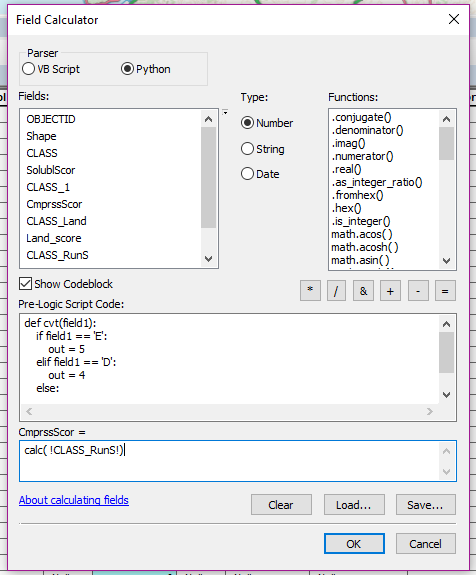Works perfectly. Thank you.
``````byMVP Esteemed Contributor

null values are usually numbers that are unlikely to occur

for example, yours appear to be nominal classes ie class 1 is meaningless when comparing it to class 2, they are just different, the number doesn't mean anything.  A value of -9 or -99 or -999 or -9999 plus others go back a looooong time as null values in that sense.

Similarly if your data represented counts, in otherwords 1 and 2 now have meaning, 1 means you saw one ..., 2 means you counted two and 2 means that you counted twice as many as 1.  -9 isn't possible, it doesn't mean count backwards, there is no such thing as a count of -9 ... counts are either 0 or positive OR!!! if you forgot to count something, you sure don't want to put in a 0 because that would imply you 'counted' that object and there were 0 found.  -9 means, it wasn't done

Glad the Jake's worked out? or was it mine? so confusing